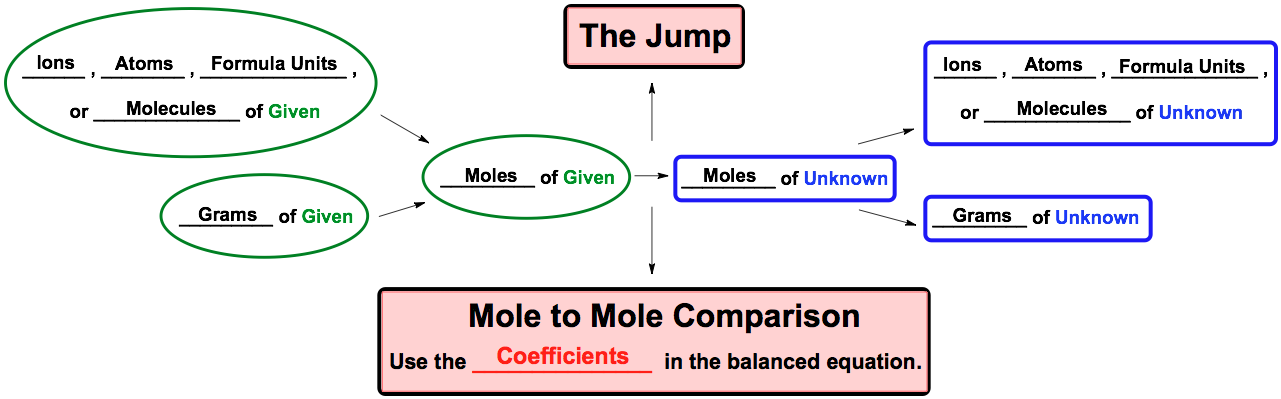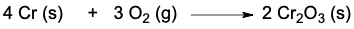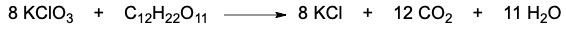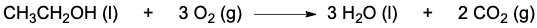Clutch Prep is now a part of Pearson
Ch.3 - Chemical ReactionsWorksheetSee all chapters

# Stoichiometry

See all sections
Sections
Empirical Formula
Molecular Formula
Combustion Analysis
Combustion Apparatus
Polyatomic Ions
Naming Ionic Compounds
Writing Ionic Compounds
Naming Ionic Hydrates
Naming Acids
Naming Molecular Compounds
Balancing Chemical Equations
Stoichiometry
Limiting Reagent
Percent Yield
Mass Percent
Functional Groups in Chemistry

Stoichiometry deals with the numerical relationship between compounds in a balanced chemical equation.

###### Stoichiometry

Concept #1: Stoichiometry

Concept #2: Stoichiometric Chart

Utilize the stoichiometric chart to do all your stoichiometric calculations.Example #1: How many grams of H2O are produced when 12.3 g H2 reacts?

2 H2 (g) + 1 O2 (g) → 2 H2O (g)

Practice: The oxidation of chromium solid is represented by the following equation:How many moles of chromium (III) oxide are produced when 34.69 g Cr reacts with excess oxygen gas?

Practice: The reaction of potassium chlorate and sucrose is given below:If 2.33 x 10-7 formula units of potassium chlorate are reacted, how many grams of carbon dioxide will be produced?

Practice: If the density of ethanol, CH3CH2OH, is 0.789 g/mL, how many milliliters of ethanol are needed to produce 4.8 g of H2O in the following reaction?